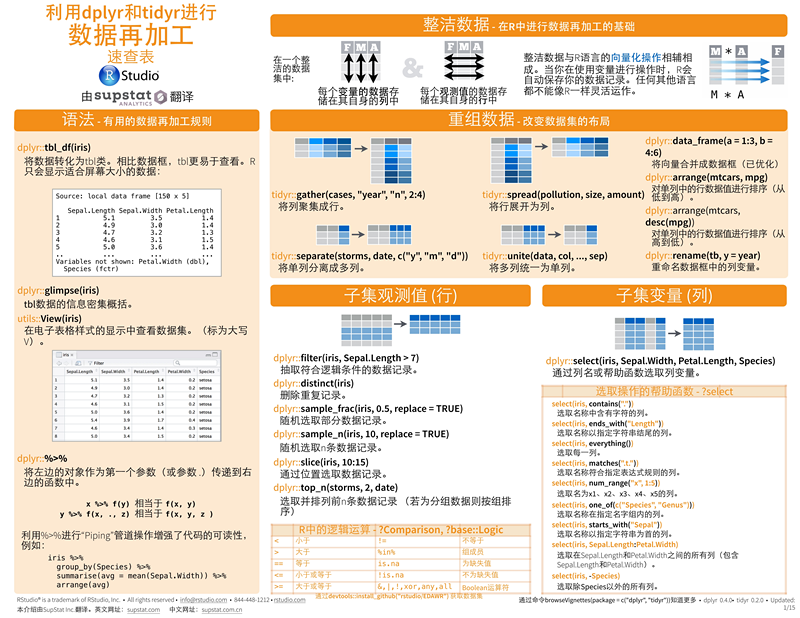# dplyr包：plyr包的替代者，专门面对数据框，将ddplyr转变为更易用的接口

%>%来自dplyr包的管道函数，其作用是将前一步的结果直接传参给下一步的函数，从而省略了中间的赋值步骤，可以大量减少内存中的对象，节省内存，可惜的是应用范围还不是很广。

# dplyr和data.table（易于操作数据）是R的两个高效数据处理包，这两个包有它们各自的优点。

data.table在语法灵活性和performance上面更深一筹，dplyr则在易学性和SQL语句转换方面有独到之处

dplyr很好地解决了base包中的几个数据处理的痛点，具体可以参考本系列01。

 12 arrange(mtcars, mpg) # 从低到高arrange(mtcars, desc(mpg)) # 从高到低

 123456 left_join(a, b, by="x1")right_join(a, b, by="x1")inner_join(a, b, by="x1")outer_join(a, b, by="x1")semi_join(a, b, by="x1") # 数据集a中能与数据集b匹配的记录anti_join(a, b, by="x1") # 数据集a中雨数据集b不匹配的记录

dplyr还提供了intersection、union和setdiff用于获得两个数据集的交集、并集和差集。

dplyr可以方便地对数据框进行概述，相当于ddply中的summarize。summarise(iris, avg=mean(Sepal.Length))将数据概括为单行结果输出；summarise_each(iris, funs(mean))对每一列运行概述函数。支持的概述函数有firstlastnthnn_distinctminmaxmeanmedianvarsd

dplyr可以方便地创建新变量，相当于ddply中的transform。mutate(iris, sepal=Sepal.Length+Sepal.Width)计算并添加一个或多个新列。mutate_each(iris, funs(min_rank))对每一列运行窗口函数。常见的窗口函数有row_numbermin_rankpercent_rankcumsum等。

dplyr提供了一个分组函数group_by，把分组依据相同的数据组合成行，相当于ddply中的group_vars。ungroup用于移出数据框的分组信息。配合上管道符号，可以方便地进行分组概述和分组计算。

 12 iris %>% group_by(Species) %>% summarise(...)iris %>% group_by(Species) %>% mutate(...)

## 强大的dplyr包实现数据预处理

select(): 按列变量选择
filter(): 按行名称分片
slice(): 按行索引分片
mutate(): 在原数据集最后一列追加一些数据集
summarise(): 每组聚合为一个小数量的汇总统计，通常结合gruop_by()使用
arrange(): 按行排序

#tbl对象

tbl_df(src, ...)

tbl_cube(dimensions, measures)

tbl_sql(subclass, src, from, ..., vars = attr(from, 'vars'))

#观测筛选

filter(.data, ...)

.data为tbl对象

...为观测筛选条件，类似于subset()函数中的用法，但不同的是filter()函数不能筛选某些变量。

df <- data.frame(x = c('a','b','c','a','b','e','d','f'), y = c(1,2,3,4,5,6,7,8))

df2tbl <- tbl_df(df)

filter(df2tbl,x %in% c('a','b'))

#变量选取

filter()函数只能将指定条件的观测筛选出来，并不能筛选出只关心的变量，为了弥补这个缺陷，可以使用select()函数筛选指定的变量，而且比subset()函数更灵活，而且选择变量的同时也可以重新命名变量。如果剔除某些变量的话，只需在变量前加上负号“-”。之所以说他比subset()函数灵活，是因为可以在select()函数传递如下参数：

starts_with(x, ignor.case = TRUE)#选择以字符x开头的变量

ends_with(x, ignor.case = TRUE)#选择以字符x结尾的变量

contains(x, ignor.case = TRUE)#选择所有包含x的变量

matches(x, ignor.case = TRUE)#选择匹配正则表达式的变量

num_range('x', 1:5, width = 2)#选择x01到x05的变量

one_of('x','y','z')#选择包含在声明变量中的

everything()#选择所有变量，一般调整数据集中变量顺序时使用

#将df2tbl数据集中的y变量放到x变量前

select(df2tbl,y,everything())

#筛选变量的同时，重新命名变量名

select(df2tbl, x1 = x, y1 = y)

#重命名变量

rename(tbl, newname = oldname,...)

rename(df2tbl, x1 = x, y1 = y)

#数据排序

arrange(.data, ...)

arrange()函数默认以某个变量进行升序，如需降序则desc(var_name)即可。

arrange(df2tbl, y) #默认升序

arrange(df2tbl, desc(y)) #降序操作

#数据扩展

mutate(.data, ...)

mutate(df2tbl, z = y^2 + y - 10)

transmute(df2tbl, z = y^2 + y - 10)

#数据聚合

summarize(.data, ...)

min()：返回最小值

max()：返回最大值

mean()：返回均值

sum()：返回总和

sd()：返回标准差

median()：返回中位数

IQR()：返回四分位极差

n()：返回观测个数

n_distinct()：返回不同的观测个数

first()：返回第一个观测

last()：返回最后一个观测

nth()：返回n个观测

summarize(df2tbl, max(y))

summarize(df2tbl, n())

summarize(group_by(df2tbl,x), sum(y))

#数据关连

inner_join#內连
left_join#左连
right_join#右连
full_join#全连
semi_join#返回能够与y表匹配的x表所有记录
anti_join#返回无法与y表匹配的x表的所记录
intersect(x, y): x 和 y 的交集（按行）
union(x, y): x 和 y 的并集（按行）
setdiff(x, y): x 和 y 的补集 （在x中不在y中）

df2 <- data.frame(x = c('a','b','c'), z = c('A','B','C'))

df2tbl2 <- tbl_df(df2)

inner_join(x = df2tbl, y = df2tbl2, by = 'x')

semi_join(x = df2tbl, y = df2tbl2, by = 'x')

anti_join(x = df2tbl, y = df2tbl2, by = 'x')

#数据合并

mydf1 <- data.frame(x = c(1,2,3,4), y = c(10,20,30,40))

mydf2 <- data.frame(x = c(5,6), y = c(50,60))

mydf3 <- data.frame(z = c(100,200,300,400))

bind_rows(mydf1, mydf2)

bind_cols(mydf1, mydf3)

#管道函数

inner_join(x = df2tbl, y = df2tbl2, by = 'x') %>% group_by(., z) %>% summarize(., max(y))

#连接数据库数据

src_mysql(dbname, host = NULL, port = 0L, user = "root", password = "",

...)

tbl(src,from = '')

src为src_mysql()函数对象

from为SQL语句

src <- src_mysql('test', host = 'localhost', user = 'root', password = 'snake')

src

#获取指定表中的数据

tbl(src, from = 'diff')posted @ 2016-11-14 01:24  Little_Rookie  阅读(13219)  评论(0编辑  收藏  举报